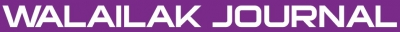### Two Dimensional Lattice Boltzmann Method for Cavity Flow Simulation

Panjit MUSIK, Krisanadej JAROENSUTASINEE

#### Abstract

This paper presents a simulation of incompressible viscous flow within a two-dimensional square cavity. The objective is to develop a method originated from Lattice Gas (cellular) Automata (LGA), which utilises discrete lattice as well as discrete time and can be parallelised easily. Lattice Boltzmann Method (LBM), known as discrete Lattice kinetics which provide an alternative for solving the Navier–Stokes equations and are generally used for fluid simulation, is chosen for the study. A specific two-dimensional nine-velocity square Lattice model (D2Q9 Model) is used in the simulation with the velocity at the top of the cavity kept fixed. LBM is an efficient method for reproducing the dynamics of cavity flow and the results which are comparable to those of previous work.

#### Keywords

Cavity flow, Lattice Boltzmann Method, Lattice Gas (cellular) Automata, Incompressible Viscous flow, D2Q9 Model

PDF

#### References

Succi S. The Lattice Boltzmann Equation for Fluid Dynamics and Beyond. Oxford University Press, 2001.

Guo Z Shi B Wang N. Lattice BGK model for incompressible Navier-Stokes equation. J Com Phys 2000; 165: 288-306.

Qian Y d’Humieres D Lallemand P. Lattice BGK models for Navier-Stokes equation. Euro Phys Let 1992; 17: 479-84.

Satofuka N Nishioka T. Parallelisation of Lattice Boltzmann method for incompressible flow computation. Com Mech 1999; 23: 164-71.

Mei R Luo LS Shyy W. An accurate curved boundary treatment in the Lattice Boltzmann Method. Com Phys 1999; 155: 307-30.

Chen H Chen S Matthaeus WH. Recovery of the Navier-Stokes equation using a Lattice-Gas Boltzmann method. Phys Rev A 1992; 45: 5339-42.

Chen S Doolen GD. Lattice Boltzmann method for fluid flows. Ann Rev Fluid Mec 1998; 30: 329-64.

Wolf-Gladrow DA. Lattice-Gas Cellular Automata and Lattice Boltzmann Models: An Introduction, Lecture Notes in Mathematics, Springer-Verlag, 2000.

Jaroensutasinee K Jaroensutasinee M. Parallel construction of poincare surface of section method on WAC16P4 cluster. Proceedings of the 6th National Computer Science and Engineering Conference 2002, 217-22.

Maeder RE. Computer Science with Mathematica. CUP (USA), 2000.

Wolfram S. The Mathematica Book. CUP and Wolfram Media, 1999.

Bhatnagar PL Gross EP Krook M. A model for collision processes in gases. 1. Small amplitude processes in harged and neutral one-component systems. Phys Rev 1954; 94: 511-25.

Succi S. The Lattice Boltzmann Equation for Fluid Dynamics and Beyond (Numerical Mathematics and Scientific Computation). Oxford University Press, 2001.

Hou SL Zou Q Chen SY Doolen G Cogley AC. Simulation of cavity flow by the Lattice Boltzmann method. J Com Phys 1995; 118: 329-47.

### Refbacks

• There are currently no refbacks.Online ISSN: 2228-835X

http://wjst.wu.ac.th

Last updated: 12 August 2019